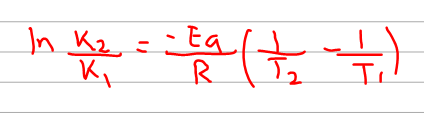# Problem: The following reaction has an activation energy of 262 kJ/mol.C4H8(g) → 2C2H4(g)At 600.0 K the rate constant is 6.1 x 10-8 s-1. What is the value  of the rate constant at 725.0 K?

###### FREE Expert Solution
87% (107 ratings)
###### FREE Expert Solution

The problem gave us the values for activation energy, 2 temperatures, and 1 rate constant k. Now it asks us another k value at a certain temperature. Based on these, we can say that we would need to use the 2 point form of the Arrhenius equation.87% (107 ratings)###### Problem Details

The following reaction has an activation energy of 262 kJ/mol.

C4H8(g) → 2C2H4(g)

At 600.0 K the rate constant is 6.1 x 10-8 s-1. What is the value  of the rate constant at 725.0 K?

What scientific concept do you need to know in order to solve this problem?

Our tutors have indicated that to solve this problem you will need to apply the Arrhenius Equation concept. You can view video lessons to learn Arrhenius Equation. Or if you need more Arrhenius Equation practice, you can also practice Arrhenius Equation practice problems.

What is the difficulty of this problem?

Our tutors rated the difficulty ofThe following reaction has an activation energy of 262 kJ/mo...as medium difficulty.

How long does this problem take to solve?

Our expert Chemistry tutor, Dasha took 5 minutes and 44 seconds to solve this problem. You can follow their steps in the video explanation above.

What professor is this problem relevant for?

Based on our data, we think this problem is relevant for Professor Blue's class at UK.